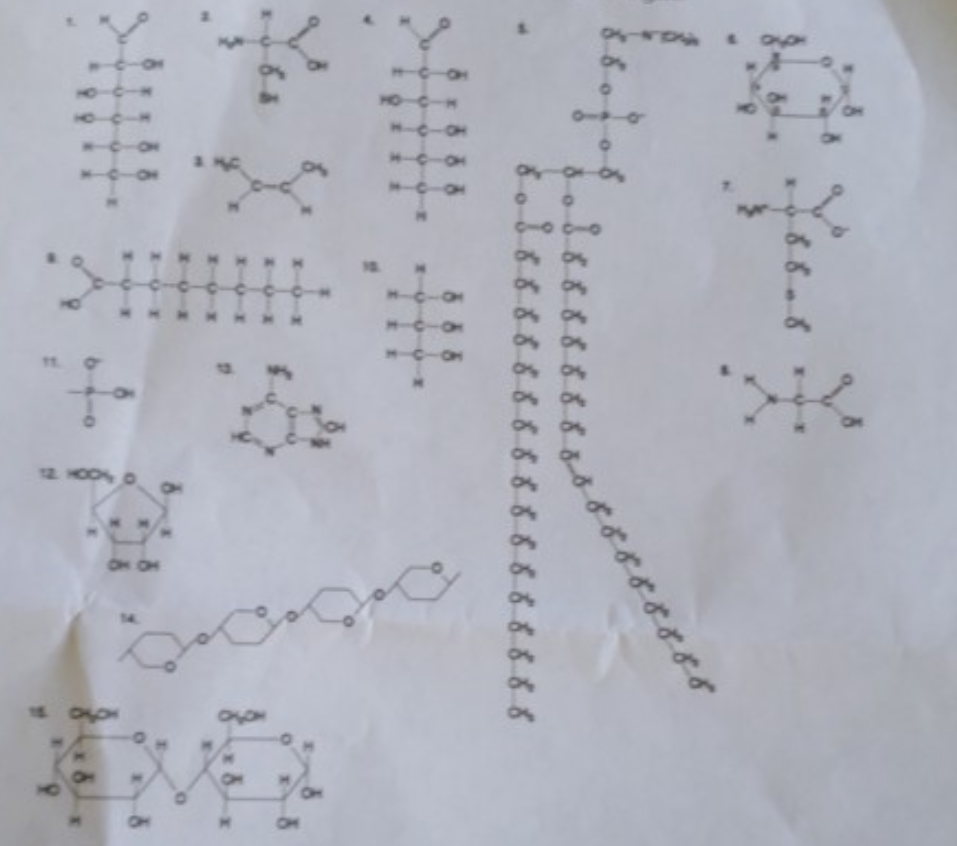# Problem: The following question is based on the 15 molecules illustrated in the figureUsing the above figure, a phospholipid would be formed as a result of a dehydration reaction between ______A) two molecules of 9, one molecule of 10 and one molecule of 11B) one molecules of 9, one molecule of 10 and two molecules of 11C) one molecule of 10 and three molecules of 9 D) one molecule of 5 and one molecule of 10

###### FREE Expert Solution

Phospholipids: major component of membranes###### Problem Details

The following question is based on the 15 molecules illustrated in the figureUsing the above figure, a phospholipid would be formed as a result of a dehydration reaction between ______

A) two molecules of 9, one molecule of 10 and one molecule of 11

B) one molecules of 9, one molecule of 10 and two molecules of 11

C) one molecule of 10 and three molecules of 9

D) one molecule of 5 and one molecule of 10

Frequently Asked Questions

What scientific concept do you need to know in order to solve this problem?

Our tutors have indicated that to solve this problem you will need to apply the Lipids concept. You can view video lessons to learn Lipids. Or if you need more Lipids practice, you can also practice Lipids practice problems.